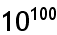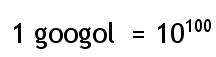Home Math Calculators Math  Flashcards Math Games Math  Homework Math Lessons Math Terms Math Worksheets Math Tools
You Are In Math Glossary of Terms

# Googol

Googol is the large number, that is, the digit 1 followed by one hundred zeros.

Example :